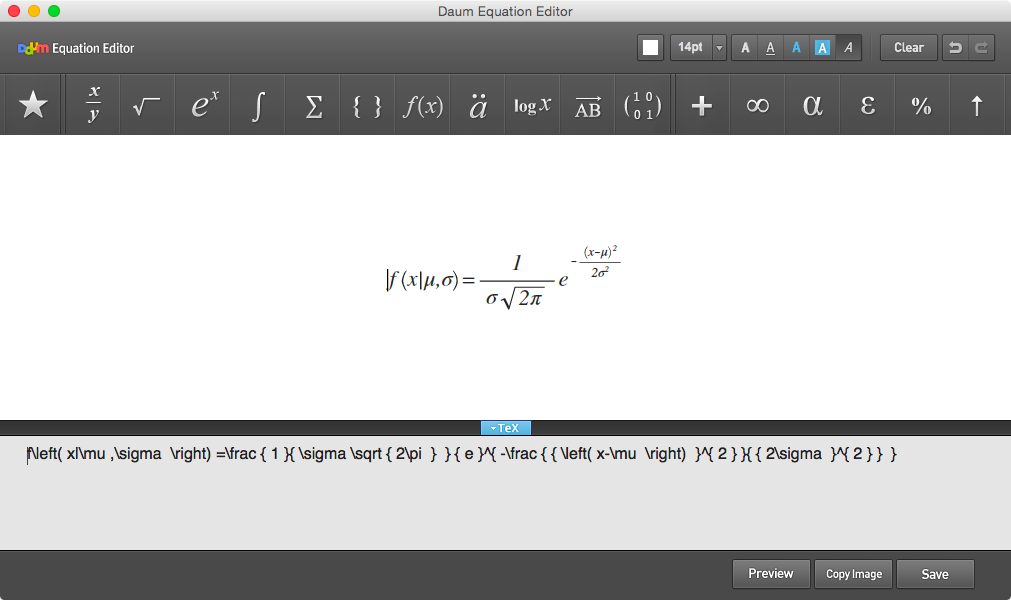# Math Equations

Occasionally you will need to type equations in homework and labs. R Markdown supports LaTeX style equations using the MathJax javascript library. I do not expect you to learn LaTeX for this course. Instead, I recommend using the free application [Daum Equation Editor](). It availabe online, as a Google Chrome Extension, or as a standalone Mac Application.

## Creating Equations with Daum Equation Editor

Occasionally you will need to type equations in homework and labs. R Markdown supports LaTeX style equations using the MathJax javascript library. I do not expect you to learn LaTeX for this course. Instead, I recommend using the free application [Daum Equation Editor](). It availabe online, as a Google Chrome Extension, or as a standalone Mac Application. With the editor, you can enter quations using menus.Once done, copy and paste the LaTeX code at teh bottom into your R Markdown file between two dollar signs (i.e. \$), and the quation will be rendered by the web browser.

$$f\left( x|\mu ,\sigma \right) =\frac { 1 }{ \sigma \sqrt { 2\pi } } { e }^{ -\frac { { \left( x-\mu \right) }^{ 2 } }{ { 2\sigma }^{ 2 } } }$$